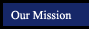Section Title Tutorials from this book Examples to copy (in pop-up window) 5.1 Exponents 1 5 a b c d e f g h i j 5.2 More on Exponents 5.3 Mutiplying and Dividing Monomials a b c d e 5.4 Scientific Notation 5.5 Polynomials 5.6 More on Polynomials a b c d e 5.7 Addition of Polynomials a b c d e 5.8 Subtraction of Polynomials 5.9 Multiplication of Monomials and Binomials a b c 5.10 Multiplying Binomials: Special Products 1 3 5 5.11 Multiplying Polynomials 5.12 Reasoning Strategies: Make a Table,Look for a Pattern
 Suggested Assignments Section Topic Pages Exercises 5.1 Exponents 204 5.2 More with Exponents 209 5.3 Mutiplying and Dividing Monomials 214 5.4 Scientific Notation 217 5.5 Polynomials 221 5.6 More on Polynomials 226 5.7 Addition of Polynomials 231 5.8 Subtraction of Polynomials 236 5.9 Multiplication of Monomials and Binomials 240 5.10 Multiplying Binomials: Special Products 245 5.11 Multiplying Polynomials 249 5.12 Reasoning Strategies: Make a Table, Look for a Pattern 254 Chapter 5 Wrap Up 256 Chapter 5 Assessment 259

Online Scientific Calculator from Calculator.com

Math Glossary
(more sophisticated than it looks!)